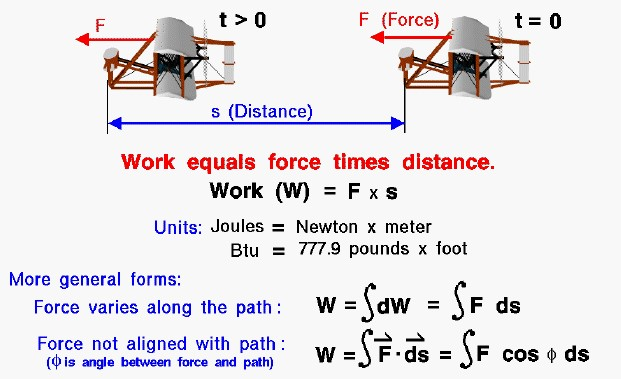# Work Equals Force Times DistanceFor scientists, work is the product of a force acting on an object times the distance that the object moves. As an example shown on the slide, the Wright 1903 aircraft is acted upon by the thrust force (F) from time (t) equals zero to some later time (t > 0) and travels some distance (s). The work (W) done on the aircraft during this time is F times s.

### W = F * s

The units of work in the metric system is the Joule, which is equal to one Newton times one meter. In the English system the unit of work is the British thermal unit (Btu) which is equal to 777.9 pounds times one foot. Notice that the units of work are the same as the units of energy. Work and energy are related to one another as we shall see in our studies of thermodynamics.

In our simple example, the force is a constant value aligned with the displacement of the aircraft. In most real applications, the force varies along the flight path in both magnitude and direction. The work is then equal to the integrated value of the component of the force in the direction of the displacement with the angle between the force and displacement given by the Greek symbol “phi”. It is important to note that work is only done on (or by) the component of the force aligned with the path. Using a cruising aircraft as another example, the lift is defined to be the force perpendicular to the flight path. Lift does no work on a cruising aircraft because the displacement is perpendicular to the force. Similarly, if an aircraft was stopped at the end of the runway with the brakes on and the engines at full throttle, the engines do no work on the aircraft because the displacement distance is zero.

The Wright brothers used their knowledge of work and power, the time rate of change of work, to determine the minimum power requirements for their 1903 engine design. Power is equal to the force acting on an object times the velocity of the object. The brothers were able to make rather accurate estimates of the drag force on their 1903 aircraft based on the flight tests of the 1902 glider and the 1901 wind tunnel results. Knowing the drag and the desired flight velocity they computed the power requirements for the engine in order to develop thrust to overcome the drag of the aircraft.

In the previous examples, we have been concerned with the work done by a force applied to a solid object. For a gas, work is done when the volume of a gas is changed at some pressure. Pressure represents a force per unit area and the volume can be related to the surface area and a distance travelled by that surface when the volume changes. (force / area * area * distance = force * distance = work). Notice that to produce work, the volume of a gas must change.

Provide feedback